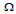# Digital Electronics - Digital Design

### Exercise :: Digital Design - General Questions

16.

A settable flip-flop's normal starting state when power is first applied to a circuit is always the ________ state.

 A. reset B. set C. toggle D. dual

Explanation:

No answer description available for this question. Let us discuss.

17.

In the automatic reset circuit for a flip-flop, how long does it take the capacitor to completely charge?

 A. 1 time constant (RC) B. 2 time constants (RC) C. 5 time constants (RC) D. 10 time constants (RC)

Explanation:

No answer description available for this question. Let us discuss.

18.

The output of a standard TTL NAND gate is used to pull an LED indicator LOW. The LED is in series with a 470-resistor. What is the current in the circuit when the LED is on?

 A. 7.02 mA B. 8.51 mA C. 10.63 mA D. 5.32 mA

Explanation:

No answer description available for this question. Let us discuss.

19.

When the inputs to a flip-flop are changing at the same time that the active trigger edge of the input clock is making its transition, this condition is called:

Explanation:

No answer description available for this question. Let us discuss.

20.

Is the propagation delay from the clock to the output for the 7476 the same as the delay from the set or reset to the output?

 A. yes B. no